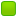###Author Topic: yo  (Read 2891 times)

0 Members and 1 Guest are viewing this topic.

####Ender

• x86
• Hero Member
••• Posts: 2398##### yo
« on: May 17, 2008, 12:00:18 am »
you need to change your forum description from this "3.141592654"

to this
$$2\int_{-1}^{1} \sqrt{1-x^2}dx$$

or at least to this "3.141592654..."

GET WTIHT HE PROGRAM.

####Joe

• B&
• Moderator
• Hero Member
••• Posts: 10330
• In Soviet Russia, text read you!##### Re: yo
« Reply #1 on: May 17, 2008, 03:15:30 am »
4 * (1 - 1/3 + 1/5 - 1/7 + 1/9 - 1/11...)

What's the proper way of writing that continuing sequence?

####Ender

• x86
• Hero Member
••• Posts: 2398##### Re: yo
« Reply #2 on: May 17, 2008, 03:39:49 am »
4 * (1 - 1/3 + 1/5 - 1/7 + 1/9 - 1/11 + ...) is more conventional

you get this from pi/4 = arctan(1), and then the sum 1 - 1/3 + 1/5 + ... is the taylor series for arctan evaluated at 1

but pi is often defined as the integral that i posted, whereas this other representation is derived

speaking of which, it's cool how cos is defined:

$$for \; -1 \leq x \leq 1 \; let \;A(x) = x\sqrt{1-x^2} + 2\int_{x}^{1} \sqrt{1-t^2}dt$$

then we define

$$\cos = A^{-1}$$

but you first have to show that A is a bijection (it's sufficient to show that it's strictly decreasing)

and then sin is defined as

$$\sin x = \sqrt{1 - \cos^2 x}$$